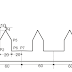MAIN PROG .

O5000
N10   G90 G94 F300 ;
N20   G00 X-10 Y0 ;
N30   G17 G01 X15 D01 ;                                 [P0]
N40   G01 Y20 ;                                                 [P1]
N50   G72.2 P5100 L3 I60 J0 ;
N60   G90 X195 Y0 ;                                         [P8]
N70   G00 X-10 ;
N80   M30 ;

SUB PROG.

O5100 N100 G91 G01 X20 ;                            [P2]
N200 G01 Y20 ;                                                [P3]
N300 G01 X10 Y20 ;                                        [P4]
N400 G01 X10 Y-20 ;                                       [P5]
N500 G01 Y-20 ;                                               [P6]
N600 G01 X20 ;                                                [P7]
N700 M99 ;
More examples..........!!!!

DESCRIPTION OF PROGRAM :-

Main prog.
O5000-  Number of main program
N10- Absolute co-ordinate command , feed rate per minute command , feed is 300
N20- Rapid command , where tool at safe position X-10 and Y0 .
N30- Slection of XY plane command , linear interpolation command , where X15 and Y0 (it means tool at position P0 )
N40- Linear interpolation command , where tool is X15 and Y20 [P1]
N50- Linear copy command , call sub program no.5100 , no. of times the operation is repeated , tool shift along X and Y axis is 60 and 0.
N60- Absolute co-ordinate command ( to cancel incremental command ), when tool at X195 and Y0.
N70- Rapid commad , where tool is return at X-10 and Y0
N80- Main program end .

Sub prog.
[note:- In these program sub program name and other first command written in same row  like; O5100 N100 G91 G01 X20 ;]

O5100- No. of sub program , N100 - Incremental co-ordinate command ( each distance count from current position)  , linear interpolation command where X20 and Y 20 .[P2]
N200- Linear interpolation command , where tool travel along y axis is 20 . [P3] { up}
N300- Liner interpolation command , where tool travel along X10 and Y20 (taper ) [P4]{up}
N400- Liner interpolation command , where tool travel along X10 and Y-20 (taper ) [P5] {down}
N500- Linear interpolation command , where tool travel along y axis is -20 . [P6] {down}
N600- Linear interpolation command , where tool travel along x axis is 20 .[P7]
N700- Sub prog. end

Fanuc G72.2 linear copy program for cnc milling operationReviewed by harshal on August 13, 2018 Rating: 5